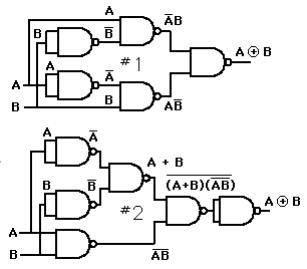Competitions

# XOR SumYou will be given a list of q (1q100000) instructions.

If the instructions is to "**insert n**", insert n into the list of numbers (there may be duplicates).

If the instruction is to "print" - print the XOR sum of the largest k (1kq) elements in the list (or, if the list contains less than k elements, the XOR sum of all elements in the list).

XOR sum of a list of numbers is the result of XOR-ing all of them. XOR can be applied to two integers using the built in ^ *operator in most languages (or *xor in Haskell (Pascal)).

Note that XOR function has some useful properties, among them that if n ^ m = x then n = x ^ m and m = x ^ n.

#### Input

First line contains the number t (1t30) of test cases. Each test case start with a line containing two integers q and k (1q, k100000). Following are q lines containing one instruction each.

Instructions are in either of the following two forms:

insert n

or

print

n is a non-negative integer less than 231.

#### Output

For each print statement output the XOR sum of (at most) k largest elements in the current list. Note that the list is cleared between test cases.

Time limit 1 second
Memory limit 64 MiB
Input example #1
1
5 2
insert 1
insert 2
print
insert 3
print

Output example #1
3
1

Author Hichem Zakaria Aichour
Source ACM ICPC CCPC 2013